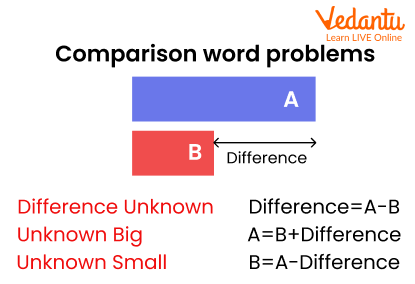Courses
Courses for Kids
Free study material
Free LIVE classes
More

# Additive Comparison – Definition with Solved ExamplesLIVE
Join Vedantu’s FREE Mastercalss

As students progress through higher classes, many new concepts are introduced to them. In mathematics, children may start solving word problems, which get more and more challenging in higher grades. These word problems are of two types: additive and multiplicative.

The additive comparison includes word problems that compare two amounts by asking or telling how much more (or less) is one amount than the other. To solve additive comparison problems, students need to first form an equation in terms of variables such as ‘x’ and ‘y’.Two verbal statements are used in additive comparison problems such as ‘how much more? ‘x more’, ‘how much less?’, ‘x less’, etc. Remember that the identifying feature of additive comparison is that it usually includes words like ‘more’ and ‘less’.

Here is an example of an additive comparison statement.

Preeti has 6 chocolates. Inayat has 4 more than her. How many chocolates does Inayat have?

## Solved Examples

Example 1: John has 18 pencils. Peter has 5 less than him. How many pencils does Peter have?

Solution: Number of pencils John has = 18.

Now, according to the question, Peter has 5 pencils less than John.

So, the number of pencils that Peter has = 5 pencils less than John = 18 − 5 = 13.

Therefore, Peter has 13 pencils.

Example 2: Sylvia has eight apples. Bill has twelve apples. How many more apples does Bill have than Sylvia?

Solution: Number of apples Sylvia has = 8

Number of apples Bill has = 12

So, the number of apples Bill has more than Sylvia = 12 − 8 = 4

Therefore, Bill has 4 more apples than Sylvia.

## Conclusion

Thus, additive comparison only compares two values, generally by using the words ‘more’ and ‘less’. Once students are able to form equations from the statements, they can solve any question of additive comparison quite easily.

For more easy and more detailed explanations on different Maths topics, head to our website and have an edge over your peers!

Last updated date: 01st Oct 2023
Total views: 139.2k
Views today: 3.39k

## FAQs on Additive Comparison – Definition with Solved Examples

1. What is the difference between additive comparison and multiplicative comparison?

Students can easily distinguish between the two types:

• The additive comparison focuses on comparing two quantities by highlighting the difference between them using the words more or less. You can ask the question, "How many more?" or "How much less?"

• Multiplicative comparison, on the other hand, focuses on comparing two quantities by telling how much one quantity is the specified number of times larger or smaller than the other. You can ask the question, "How many times as much?" or "How many times as many?"

2. What is the significance of teaching additive comparison?

Word problems on additive comparison are not only relevant to students for passing Maths exams but are also important in daily lives as well. A good understanding of the concept will enable children to do calculations in real-life situations easily. They will be able to quickly form an equation in their minds upon hearing any statement that requires kids to find the quantity of a thing; for example, supermarkets will provide the best practice of comparison statements to students.